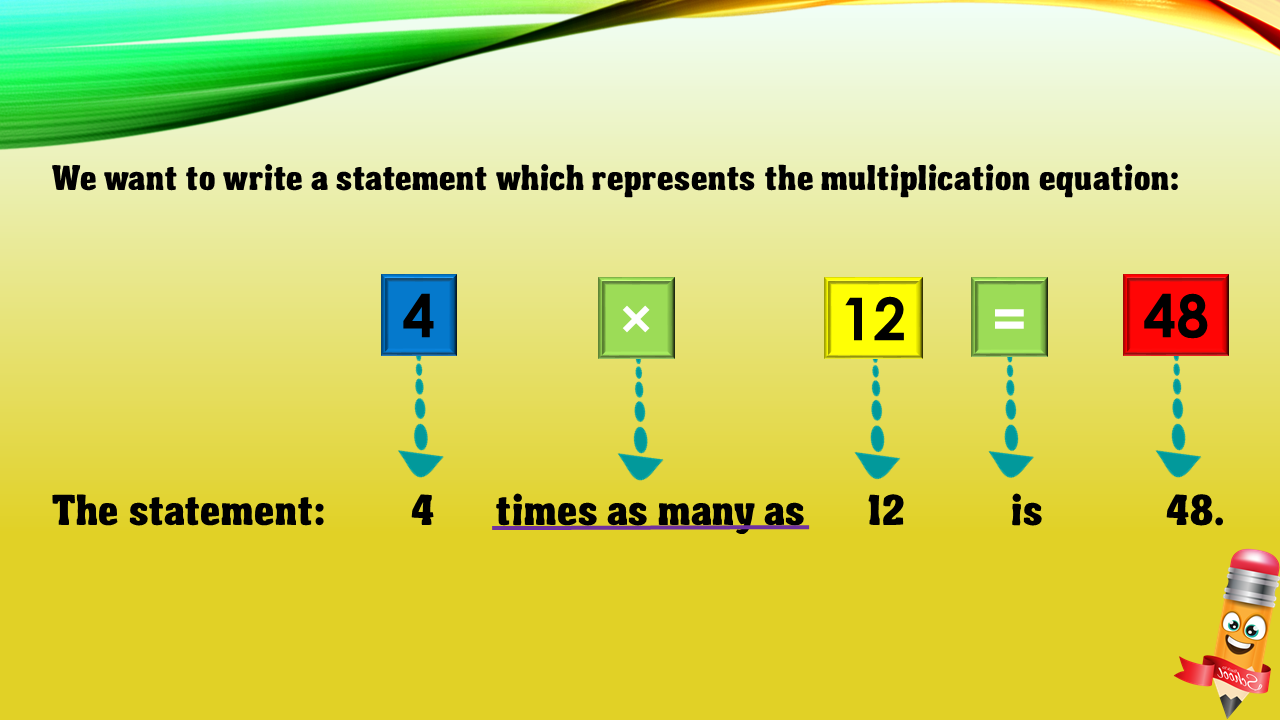1
visibility

Choose one statement which represents the equation:
4 × 12 = 48 ?

• A

4 times as many as 12 is 48.

• B

4 more than 12 is 48.

• C

12 less than 48 is 4.

• D

12 times as many as 48 is 4.